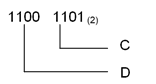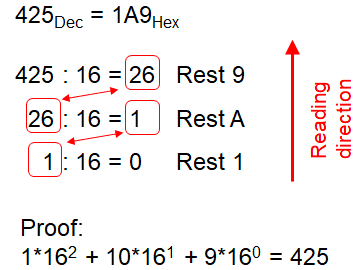# Conversion between number systems

## Conversion between number systems

### Relation between Dual code and Hexadecimal code:

When we compare the binary coded number ‘1100 1101’ with the number in hex ‘CD’, we recognize, that always 4 binary digits build a hex digit. The reason is that with 4 binary numbers exactly 16 numbers can be displayed (from 0 to F).Relation between Dual code and Hexadecimal code

### Decimal to Binary

Decimal numbers can be converted to binary by repeated division of the number by 2 while recording the remainder. We explain it with an example:From Decimal to Binary

### Decimal to Hexadecimal

Decimal numbers can be converted to Hex by repeated division of the number by 16 while recording the remainder. Let’s take an example to see how this works.